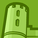Announcements
The way to achieve your own success is the willingness to help somebody else. Go for it!
cancel
Showing results for
Did you mean:Creator

## Bar Chart don't show proper graph

I have created a bar chart with the value list:

ValueList('CY', 'LY')

where CY stands for Current Year and LY stands for Last Year.

for values the below formula is used:

if(ValueList('CY', 'LY')='CY',

SUM(AGGR(if((\$(vSalesLYYTD))>0 and (\$(vSalesYTD))>0, (\$(vSalesYTD)),0),[Unit Key])),

if(ValueList('CY', 'LY')='LY',

SUM(AGGR(if((\$(vSalesLYYTD))>0 and (\$(vSalesYTD))>0, (\$(vSalesLYYTD)),0),[Unit Key]))

)

)

where vSalesLY is:

Sum({\$<[Year] = {"\$(=Year(Today())-1)"} >} [Sales])

and vSalesCY is:

Sum({\$<[Year] = {"\$(=Year(Today()))"} >} [Sales])

The graph is not showing properly. The first bar shows the rights size and figures but for the second bar it just shows zero.

If I switch the values for CY and LY; again the first bar shows the right values whereas the second bar is zero.

10 RepliesCreator
Author

there are no dimensions. Its a value list.Tags
Community Browser